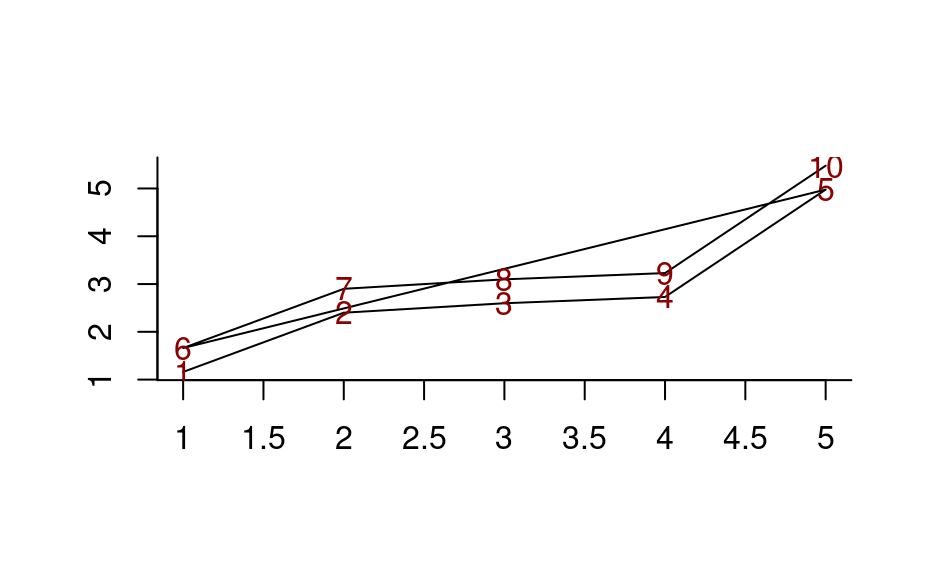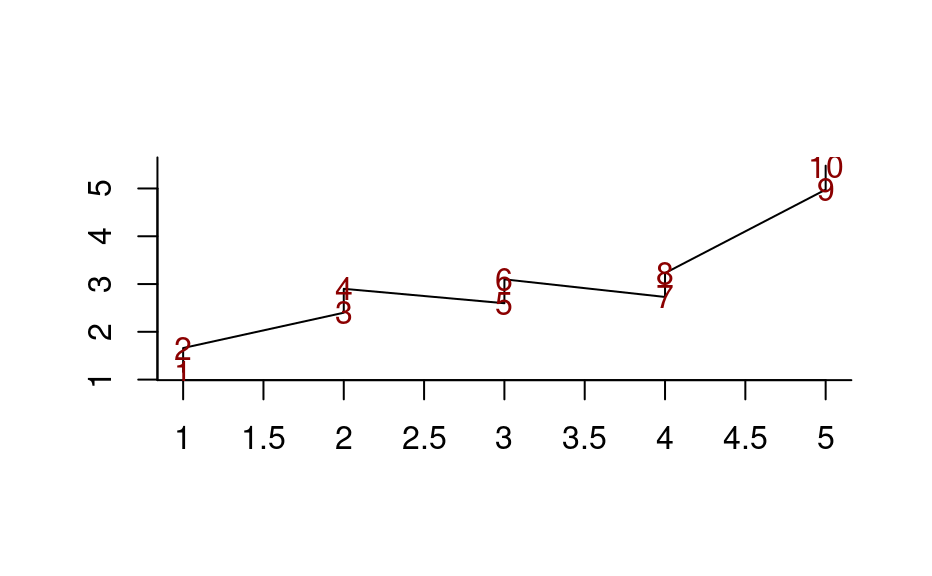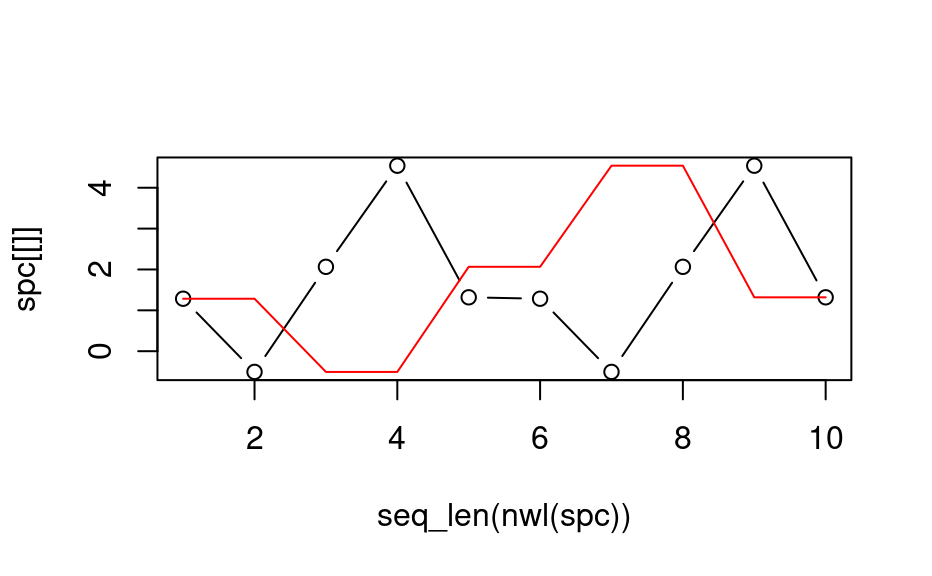These hyperSpec functions are deprecated and not maintained any more. You should not use these. Currently they are present due to back-compatibility reasons and will be removed in the next release of the package. Please, use the suggested alternative functions instead.

_____________

Rearranges the hyperSpec object so that the wavelength vector is in increasing (or decreasing) order.

The wavelength vector is sorted and the columns of the spectra matrix are rearranged accordingly.

orderwl(x, na.last = TRUE, decreasing = FALSE)

Arguments

x

The hyperSpec object.

na.last, decreasing

Handed to base::order().

Value

A hyperSpec object.

C. Beleites

Examples

## Example 1: different drawing order in plot_spc
spc <- new("hyperSpec", spc = matrix(rnorm(5) + 1:5, ncol = 5))
spc <- cbind(spc, spc + .5)

plot_spc(spc)
text(wl(spc), spc[[]], as.character(1:10), col = "darkred")spc_sorted <- orderwl(spc)
#> Warning: Function 'orderwl' is deprecated.
plot_spc(spc_sorted)
text(wl(spc_sorted), spc_sorted[[]], as.character(1:10), col = "darkred")## Example 2
spc <- new("hyperSpec", spc = matrix(rnorm(5) * 2 + 1:5, ncol = 5))
spc <- cbind(spc, spc)

plot(seq_len(nwl(spc)), spc[[]], type = "b")
spc[[]]
#>             1         2        3        4        5        1         2        3
#> [1,] 3.273145 0.2421674 4.116439 3.252164 6.387484 3.273145 0.2421674 4.116439
#>             4        5
#> [1,] 3.252164 6.387484

spc_sorted <- orderwl(spc)
#> Warning: Function 'orderwl' is deprecated.